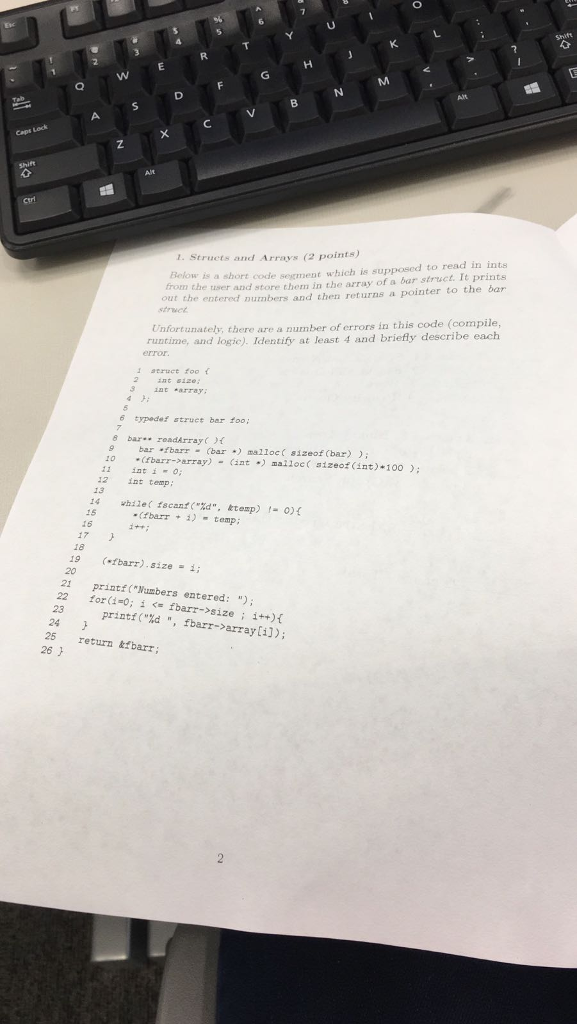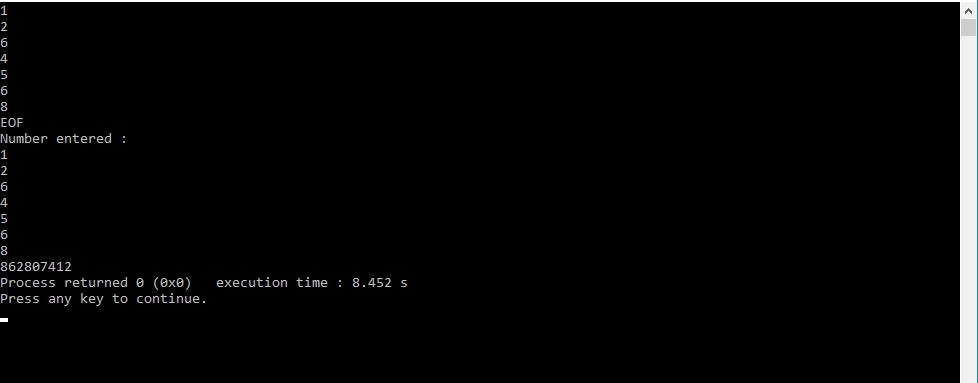# Question & Answer: Structs and Arrays Below is a short code segment which is supposed to read in ints from the user and store them in the array of a bar struct. It prints out…..Structs and Arrays Below is a short code segment which is supposed to read in ints from the user and store them in the array of a bar struct. It prints out the entered numbers and then returns a pointer to the bar struct. Unfortunately, there are a number of errors in this code (compile, runtime, and logic). Identify at least 4 and briefly describe each error. 1 struct foo { 2 int size: 3 int *array: 4 } 5 6 typedef struct bar foo: 7 8 bar** readArray () { 9 bar *fbarrr = (bar *) malloc (sizeof(bar)): 10 *(fbarr – > array) = (in *) malloc (sizeof(int) * 100): 11 int i = 0: 12 int temp: 13 14 while (fscanf(“%d”, &temp) t = 0) { 15 *(fbarr + i) = temp: 16 i++: 17 } 18 19 (*fbarr).size = i: 20 21 printf(“Numbers entered: “): 22 for (i = 0: i size: i++_ { 23 printf(“%d”, fbarr – > array[i]): 24 } 25 return &fbarr: 26 }

Don't use plagiarized sources. Get Your Custom Essay on
Question & Answer: Structs and Arrays Below is a short code segment which is supposed to read in ints from the user and store them in the array of a bar struct. It prints out…..
GET AN ESSAY WRITTEN FOR YOU FROM AS LOW AS \$13/PAGE

Solution :

line 6 : typedef struct bar foo;

this statement will replace “foo” with “struct bar” in the code.

Note that there is no struct bar .

so correct syntax is : typedef struct foo bar

–> if struct name is bar then define struct as :

struct bar{

int size;

int *array;

}

line 10 : *(fbarr->array) = (int *)malloc(sizeof(int)*100);

here memory should be assigned to pointer array.

correct : (fbarr->array) = (int *)malloc ((sizeof(int))*100);

line 14: fscanf is used to read from file.

So file pointer has to be there .

here use scanf to read from console.

line 15 : *(fbarr+i)= temp;

this can not be done.

Here int value can not be added to a struct pointer.

correct : (fbarr->array)[i] = temp;

this will store values in array .

Sample output for this program is given below :